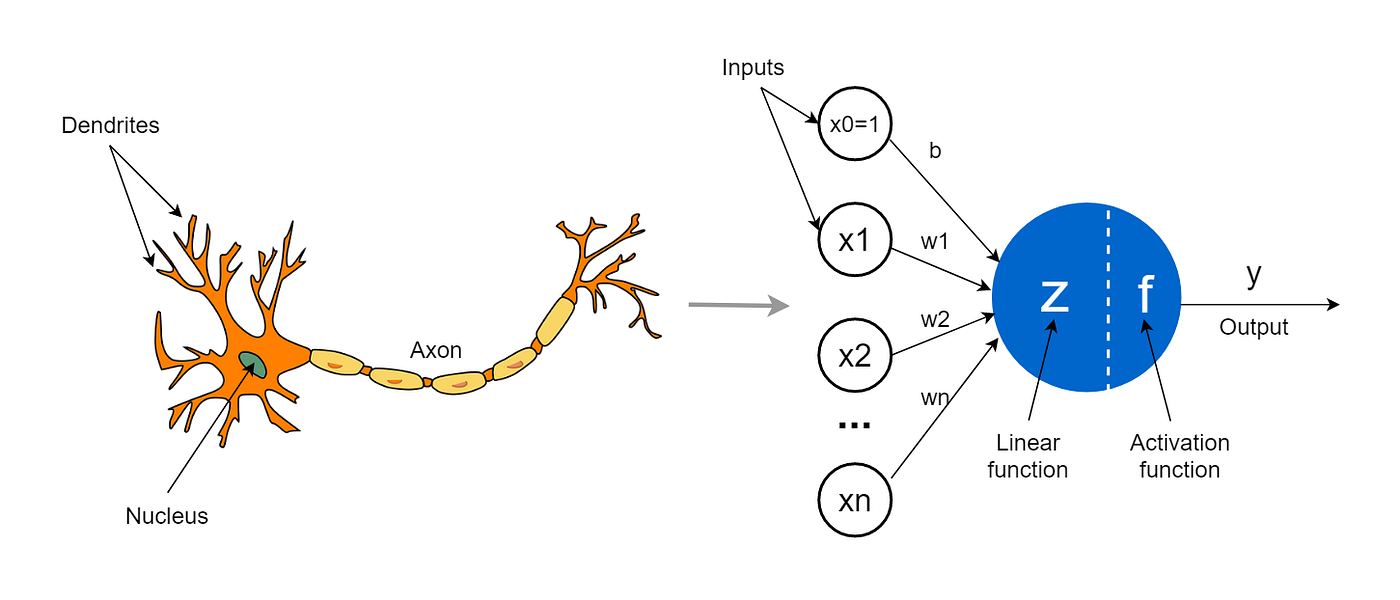# What is Deep Learning## Overview

Deep Learning

a type of machine learning based on artificial neural networks in which multiple layers of processing are used to extract progressively higher level features from data.

Machine Learning

development of computer systems that can learn to more accurately predict the outcomes without following explicit instructions, by using algorithms and statistical models to draw inferences from patterns in data.

### Differences between Deep Learning and Machine Learning

#### Machine Learning

• uses algorithms to parse data, learn from that data, and make informed decisions based on what it has learned.

• needs a human to identify and hand-code the applied features based on the data type. | tries to learn features extraction and representation as well.

• tend to parse data in parts, then combined those into a result (e.g. first number plate localization and then recognition).

• requires relatively less data and training time

#### Deep learning

• structures algorithms in layers to create an “artificial neural network” that can learn and make intelligent decisions on its own.

• tries to learn features extraction and representation as well.

• Deep learning systems look at an entire problem and generate the final result in one go (e.g. outputs the coordinates and the class of object together).

• requires a lot more data and training time

## Applications Of Machine Learning/Deep Learning

• Email spam detection

• Fingerprint / face detection & matching (e.g., phones)

• Web search (e.g., DuckDuckGo, Bing, Google)

• Sports predictions

• Credit card fraud

• Stock predictions

## Broad categories of Deep learning## Perceptron

### Definition

Simplest artificial neuron that takes binary inputs and based on their weighted sum reaching a threshold, generates a binary output.

### Artificial neurons

• Takes in multiple inputs and learns what should be the appropriate output

• Essentially a mathematical function where the weights multiplied with the inputs are learnable

• Acts like a logic gate but the operation performed adjusts according to the data• connect them in a network to create an artificial brain(let)

### History of the Perceptron

• Invented in 1957 by Frank Rosenblatt to binary classify an input data.

• An attempt to replicate the process and ability of human nervous system.

#### A Biological Neuron#### McCulloch & Pitts Neuron Model### Computational Model of a Biological Neuron### Terminology

• Net input $$=$$ weighted inputs, $$z$$

• Activations = activation function(net input); $$a=\sigma(z)$$

• Label output $$=$$ threshold(activations of last layer); $$\hat{y}=f(a)$$

Special cases:

• In perceptron: activation function = threshold function

• In linear regression: activation $$=$$ net input $$=$$ output

$\sigma\left(\sum_{i=1}^m x_i w_i+b\right)=\sigma\left(\mathbf{x}^T \mathbf{w}+b\right)=\hat{y}$

Often more convenient notation: define bias unit as $$w_0$$ and prepend a 1 to each input vector as an additional feature value

$\sigma\left(\sum_{i=0}^m x_i w_i\right)=\sigma\left(\mathbf{x}^{\top} \mathbf{w}\right)=\hat{y}$

### Perceptron Learning Algorithm

Let $$\mathcal{D}=\left(\left\langle\mathbf{x}^{}, y^{}\right\rangle,\left\langle\mathbf{x}^{}, y^{}\right\rangle, \ldots,\left\langle\mathbf{x}^{[n]}, y^{[n]}\right\rangle\right) \in\left(\mathbb{R}^m \times\{0,1\}\right)^n$$

1. Initialize $$\mathbf{w}:=0^m \quad$$ (assume notation where weight incl. bias)

2. For every training epoch:

• For every $$\left\langle\mathbf{x}^{[i]}, y^{[i]}\right\rangle \in \mathcal{D}$$ :

1. $$\hat{y}^{[i]}:=\sigma\left(\mathbf{x}^{[i] \top} \mathbf{w}\right)$$

2. err $$:=\left(y^{[i]}-\hat{y}^{[i]}\right)$$

3. $$\mathbf{w}:=\mathbf{w}+e r r \times \mathbf{x}^{[i]}$$

#### Vectorization in Python

Running Computations is a Big Part of Deep Learning!

import torch

def forloop(x, w):
z = 0.
for i in range(len(x)):
z += x[i] * w[i]
return z

def listcomprehension(x, w):
return sum(x_i*w_i for x_i, w_i in zip(x, w))

def vectorized(x, w):
return x.dot(w)

x, w = torch.rand(1000), torch.rand(1000)

%timeit -r 10 -n 10 forloop(x, w)

%timeit -r 10 -n 10 listcomprehension(x, w)

%timeit -r 10 -n 10 vectorized(x, w)

9.08 ms ± 46.8 µs per loop (mean ± std. dev. of 10 runs, 10 loops each)

7.23 ms ± 65.5 µs per loop (mean ± std. dev. of 10 runs, 10 loops each)
The slowest run took 10.90 times longer than the fastest. This could mean that an intermediate result is being cached.
6.93 µs ± 10.1 µs per loop (mean ± std. dev. of 10 runs, 10 loops each)


### Perceptron Pytorch Implementation

#### Label data

import torch
import matplotlib.pyplot as plt

c1_mean , c2_mean = -0.5 , 0.5

c1 = torch.distributions.uniform.Uniform(c1_mean-1,c1_mean+1).sample((200,2))
c2 = torch.distributions.uniform.Uniform(c2_mean-1,c2_mean+1).sample((200,2))
features = torch.cat([c1,c2], axis=0)

labels = torch.cat([torch.zeros((200,1)), torch.ones((200,1))], axis = 0)
data = torch.cat([features, labels],axis=1)

X, y = data[:, :2], data[:, 2]
y = y.to(torch.int)

print('X.shape:', X.shape)
print('y.shape:', y.shape)

X_train, X_test = X[:300], X[100:]
y_train, y_test = y[:300], y[100:]

# Normalize (mean zero, unit variance)
mu, sigma = X_train.mean(axis=0), X_train.std(axis=0)
X_train = (X_train - mu) / sigma
X_test = (X_test - mu) / sigma

plt.scatter(X_train[y_train==0, 0], X_train[y_train==0, 1], label='class 0', marker='o')
plt.scatter(X_train[y_train==1, 0], X_train[y_train==1, 1], label='class 1', marker='s')
plt.title('Training set')
plt.xlabel('feature 1')
plt.ylabel('feature 2')
plt.xlim([-3, 3])
plt.ylim([-3, 3])
plt.legend()
plt.show()

plt.scatter(X_test[y_test==0, 0], X_test[y_test==0, 1], label='class 0', marker='o')
plt.scatter(X_test[y_test==1, 0], X_test[y_test==1, 1], label='class 1', marker='s')
plt.title('Test set')
plt.xlabel('feature 1')
plt.ylabel('feature 2')
plt.xlim([-3, 3])
plt.ylim([-3, 3])
plt.legend()
plt.show()

X.shape: torch.Size([400, 2])
y.shape: torch.Size()#### Train and evaluate

import torch
device = torch.device("cuda:0" if torch.cuda.is_available() else "mps")

class Perceptron:
def __init__(self, num_features):
self.num_features = num_features
self.weights = torch.zeros(num_features, 1,
dtype=torch.float32, device=device)
self.bias = torch.zeros(1, dtype=torch.float32, device=device)

self.ones = torch.ones((1, 1), device=device)
self.zeros = torch.zeros((1, 1), device=device)

def forward(self, x):
linear = torch.mm(x, self.weights) + self.bias
predictions = torch.where(linear > 0., self.ones, self.zeros)
return predictions

def backward(self, x, y):
predictions = self.forward(x)
errors = y - predictions
return errors

def train(self, x, y, epochs):
for e in range(epochs):

for i in range(y.shape):
errors = self.backward(x[i].reshape(1, self.num_features), y[i]).reshape(-1)
self.weights += (errors * x[i]).reshape(self.num_features, 1)
self.bias += errors

def evaluate(self, x, y):
predictions = self.forward(x).reshape(-1)
accuracy = torch.sum(predictions == y).float() / y.shape
return accuracy

ppn = Perceptron(num_features=2)

X_train_tensor = torch.tensor(X_train, dtype=torch.float32, device=device)
y_train_tensor = torch.tensor(y_train, dtype=torch.float32, device=device)

ppn.train(X_train_tensor, y_train_tensor, epochs=5)

print('Model parameters:')
print('Weights: %s' % ppn.weights)
print('Bias: %s' % ppn.bias)

X_test_tensor = torch.tensor(X_test, dtype=torch.float32, device=device)
y_test_tensor = torch.tensor(y_test, dtype=torch.float32, device=device)

test_acc = ppn.evaluate(X_test_tensor, y_test_tensor)
print('Test set accuracy: %.2f%%' % (test_acc*100))